High School Physics : Electricity and Magnetism

Example Questions

Example Question #936 : High School Physics

What is the main difference between electrical and gravitational forces?

Gravitational forces are always attractive but electrical forces can be attractive or repulsive

Gravitational forces obey the inverse square law and electrical forces do not

Electrical forces obey the inverse square law and gravitational forces do not

Electrical forces attract and gravitational forces repel

Gravitational forces are always attractive but electrical forces can be attractive or repulsive

Explanation:

Electric forces can be attractive or repulsive because charges may be positive or negative. In the case for gravitational forces, there are only attractive forces because mass is always positive.

Example Question #937 : High School Physics

What is the magnitude of force a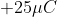charge exerts on a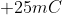charge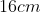away?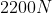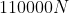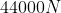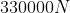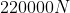Explanation:

We can calculate this using Coulomb’s Law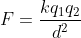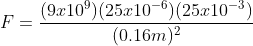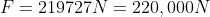Example Question #938 : High School Physics

When the magnitude of two interacting charges is increased by a factor of 2, the electrical forces between these charges is __________.

reduced by a factor of 4

reduced by a factor of 2–√

doubled

reduced by a factor of 3

Explanation:

In Coulomb's law, an increase in both interacting charges will cause an increase in the magnitude of the electrical force between them. Specifically if the magnitude of both interacting charges is doubled, this will quadruple the electrical force.

Example Question #939 : High School Physics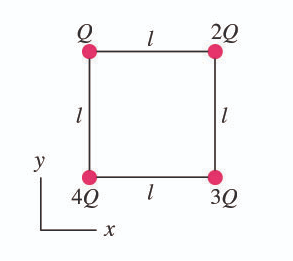At each corner of square of sidethere are point charges of magnitude Q, 2Q, 3Q and 4Q.  Determine the magnitude and direction of the force on the charge 3Q.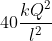away from the other charges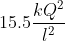away from the other chargestoward Qtoward Qaway from the other charges

Explanation:

We will need to use Coulomb’s Law to analyze the force on the 3Q charge from all the other forces.  We will then summarize the net force in the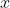and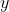direction to determine the force on the 3Q charge.

In the x-direction

Force from 4Q on 3Q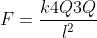Force from Q on 3Q in the x direction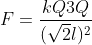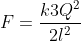Add these together in the x-direction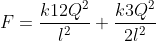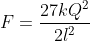In the y-direction

From from 2Q on 3Q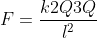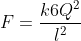Force from Q on 3Q in the y directionAdd these together in the y-direction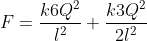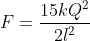Now we can find the resultant of these sides using the Pythagorean theorem.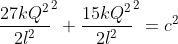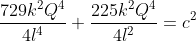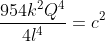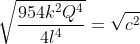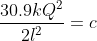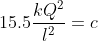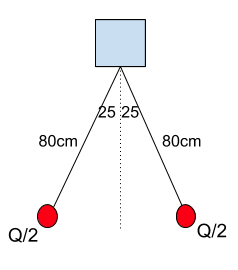Example Question #1 : Understanding Electricity

What is the maximum power consumption of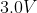portable CD player that draw a maximum of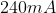of current?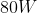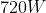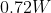Explanation:

The power in a circuit is equal to the current times the voltage.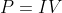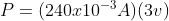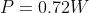Example Question #941 : High School Physics

The total amount of charge that passes through a wire’s full cross section at any point per unit of time is referred to as

Current

Voltage

Electric potential

Wattage

Current

Explanation:

Current is defined as the amount of charge that passes through a specific area of a wire in a specific interval of time.  It is measured in Amps which are Coulombs per second.

Example Question #2 : Understanding Electricity

If the resistance in a constant voltage circuit is doubled, the power dissipated by that circuit

Increase by a factor of two

Increase by a factor of four

Decrease to one fourth its original value

Decrease to one half its original value

Decrease to one half its original value

Explanation:

Decrease to one half its original value

The equation for Power isAccording to Ohm’s Law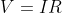We can rearrange this equation for current as this is what is changing in our circuit.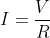We can then substitute this back into the power equation.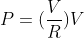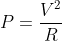If we have the original value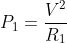and our second value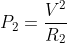We can rearrange both for the voltage and set them equal to each other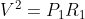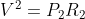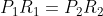So we can substitute this value in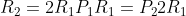And solve for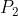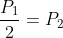Therefore the power would be cut in half.

Example Question #3 : Understanding Electricity

A current of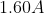flow in a wire.  How many electrons are flowing past any point in the wire per second?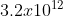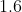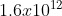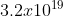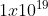Explanation:

The current is a measure of the amount of charge that passes a given point in a certain amount of time.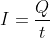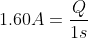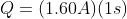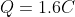To determine how many electrons are passing this point we need to look at the charge of 1 electron and do a conversion.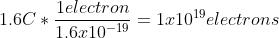Example Question #4 : Understanding Electricity

The direction of conventional current is taken to be the direction that __________________ .

positive charges would flow

negative charges would flow

positive charges would flow

Explanation:

We often think of current flowing from the top of the battery to the bottom of the battery.  The top of the battery has a higher electrical potential than the bottom of the battery and is associated as being positive.  Charges interact in such a way where like charges repel and opposite charges attract.  Since we think of the charges traveling away from the positive end of the battery and toward the negative end of the battery, this would model the motion of a positive charge (away from positive and toward negative).  Since it is not protons that move through the circuit, but rather electrons.  It is more accurate to describe the flow of electrons from the negative side of the battery to the positive side.

Example Question #5 : Understanding Electricity

Why might a circuit breaker open if you plug too many electrical devices into a single circuit?

The current becomes too high

The voltage becomes too high

The resistance becomes too high

A circuit breaker will not “trip” no matter how many electrical devices you plug into the circuit